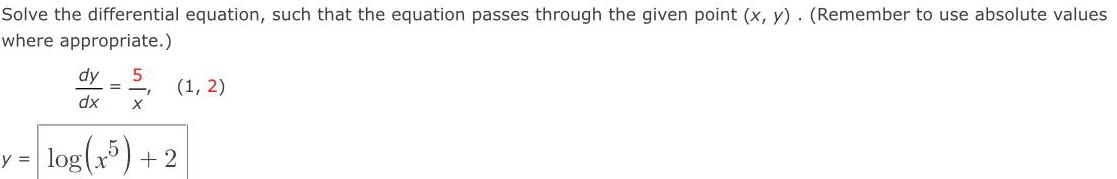Question:

# Solve the differential equation, such that the equation

Last updated: 7/28/2022Solve the differential equation, such that the equation passes through the given point (x, y). (Remember to use absolute values where appropriate.) dy/dx = 5/x (1, 2) y = log(x5) +2